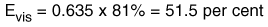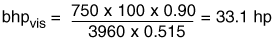### PropertiesofLiquidsSection3

Select a pump for a water capacity of 790 gpm at 109 feet head. The selection should be at or close to the maximum efficiency point for water performance. If the pump selected has an efficiency on water of 81 per cent at 790 gpm, then the efficiency for the viscous liquid will be as followsThe brake horsepower for pumping the viscous liquid will be:For performance curves of the pump selected, correct the water performance as shown below.

Instructions for Determining Pump Performance on a Viscous Liquid When Performance on Water is Known

Given the complete performance characteristics of a pump handling water, determine the performance when pumping a liquid of a specified viscosity.

From the efficiency curve, locate the water capacity (1.0 x Qw) at which maximum efficiency is obtained.

From this capacity, determine the capacities (0.6 x Qw). (0.8 x Qw) and (1.2 x Qw).

Enter the chart at the bottom with the capacity at best efficiency (1.0 x Qw), go upward to the head developed (in one stage) (Hw) at this capacity, then horizontally (either left or right) to the de-sired viscosity, and then proceed upward to the various correc-tion curves.

Read the values of (CE) and (CQ), and of (CH) for alt four capacities.

Multiply each head by its corresponding head correction factor to obtain the corrected heads. Multiply each efficiency value by (CE) to obtain the corrected efficiency values which apply at the corresponding corrected capacities.

Plot corrected head and corrected efficiency against corrected capacity. Draw smooth curves through these points. The head at shut-off can be taken as approximately the same as that for water.

Calculate the viscous brake horsepower (bhpVIS) from the formula given above.

Plot these points and draw a smooth curve through them which should be similar to and approximately parallel to the brake horsepower (bhp) curve for water.